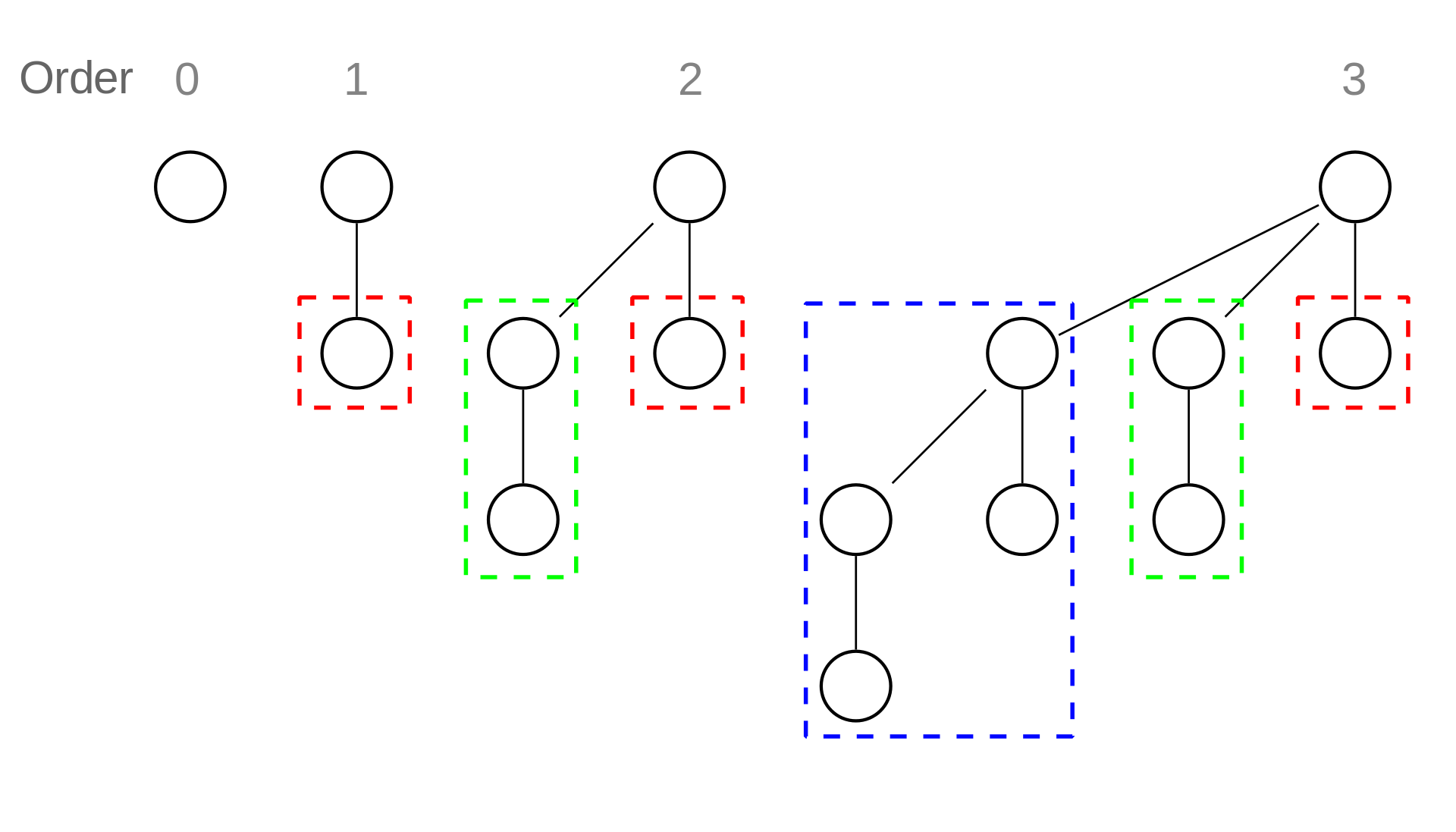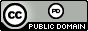시간 제한 메모리 제한 제출 정답 맞은 사람 정답 비율
1 초 (추가 시간 없음) 1024 MB 2 2 2 100.000%

## 문제Binomial trees of a binomial heap. Wikimedia, cc-by-sa

Redundant binary notation is similar to binary notation, except instead of allowing only $0$’s and $1$’s for each digit, we allow any integer digit in the range $[0, t]$, where $t$ is some specified upper bound. For example, if $t = 2$, the digit $2$ is permitted, and we may write the decimal number $4$ as $100$, $20$, or $12$. If $t=1$, every number has precisely one representation, which is its typical binary representation. In general, if a number is written as $d_l d_{l-1} \ldots d_1 d_0$ in redundant binary notation, the equivalent decimal number is $d_l\cdot2^l + d_{l-1}\cdot2^{l-1} + \cdots + d_1\cdot2^1 + d_0\cdot2^0$.

Redundant binary notation can allow carryless arithmetic, and thus has applications in hardware design and even in the design of worst-case data structures. For example, consider insertion into a standard binomial heap. This operation takes $O(\log n)$ worst-case time but $O(1)$ amortized time. This is because the binary number representing the total number of elements in the heap can be incremented in $O(\log n)$ worst-case time and $O(1)$ amortized time. By using a redundant binary representation of the individual binomial trees in a binomial heap, it is possible to improve the worst-case insertion time of binomial heaps to $O(1)$.

However, none of that information is relevant to this question. In this question, your task is simple. Given a decimal number $N$ and the digit upper bound $t$, you are to count the number of possible representations $N$ has in redundant binary notation with each digit in range $[0, t]$ with no leading zeros.

## 입력

Input consists of a single line with two decimal integers $N$ ($0 \leq N \leq 10^{16}$) and $t$ ($1 \leq t \leq 100$).

## 출력

Output in decimal the number of representations the decimal number $N$ has in redundant binary notation with each digit in range $[0, t]$ with no leading zeros. Since the number of representations may be very large, output the answer modulo the large prime $998\,244\,353$.

## 예제 입력 1

4 2


## 예제 출력 1

3


## 예제 입력 2

6 3


## 예제 출력 2

4


## 예제 입력 3

479 1


## 예제 출력 3

1


## 예제 입력 4

3846927384799 62


## 예제 출력 4

690163857


## 예제 입력 5

549755813887 2


## 예제 출력 5

1


## 출처• 문제를 만든 사람: Bryce Sandlund